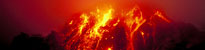# Saving data with Obspy and MiniSeed

## IntroThe next step is to save the data that our loop was producing into a file. The best option for this is using MiniSeed; a format that many seismometers use.

MiniSeed will store the figures in a file, which also contains a header that stores the metadata.

There are many different fields, which we can set in the code. We must find a few of these fields, specifically:

• start time
• number of data points
• sampling rate

## Installing ObsPy

To use the MiniSeed format, the best way is to use a library made for this: ObsPy. So we must first install it. You can use a notepad editor in root, e.g. from terminal, as long as you have an Internet connection on your Raspberry Pi.

sudo nano /etc/apt/sources.list

Add the following to the end of this sources file (the repository to the ObsPy Libraries)

deb http://deb.obspy.org wheezy main

Note: if you are using squeeze, change wheezy to squeeze — unless you don't already know what this is, in which case don't change it. Then just use the next three commands:

wget --quiet -O - https://raw.github.com/obspy/obspy/master/misc/debian/public.key | sudo apt-key add -
sudo apt-get update
sudo apt-get install python-obspy

## Programming

Now you can use ObsPy, so onto the programming.

ObsPy uses NumPy arrays for its data, this is another library, but no need to install it as it comes with ObsPy. Therefore, writing the program, we need to import these libraries, use:

import numpy
from obspy.core import Trace,Stream,UTCDateTime

And as before we need to set-up the ADC as below:

sps = 16 #samples per second

We need to declare a NumPy array in the correct format (int16), and fill it with zeros, ready to be overwritten. As below:

data=numpy.zeros([datapoints],dtype=numpy.int32)

Also we need to declare how many times we want to get samples (instead of for infinity). We want the start time for MiniSeed, using a function of ObsPy called ‘UTCDateTime’ which simply gets the time, but with as many figures as possible.

datapoints = 100
starttime=UTCDateTime()

Now we use the loop for the number of samples to go through the array filling it with values, and getting the time of each reading, as this will be used later getting sampling rate.

for x in range (datapoints):
data[x]=sample
timenow=UTCDateTime()
print sample,timenow

Now we have the array of samples for the file, we just need the header:

stats= {'network': 'UK',
'station': 'RASPI',
'location': '00',
'channel': 'BHZ',
'npts': datapoints,
'sampling_rate': '20',
'mseed' : {'dataquality' : 'D'},
'starttime': starttime}

Most of these are preset values, apart from ‘npts’ and ‘starttime’ (also sampling rate, but not yet), npts is the number of points; so 100. And ‘starttime’; we got that aswell before.

Then we put the header and data together using two ObsPy functions; Stream and Trace. These will make the data into a format that can be saved in MiniSeed. Then just save it; note that the ‘encoding’ (format for the data), and ‘reclen’; record length (the size of the file saved), should be specified.

stream.write('test.mseed',format='MSEED',encoding='INT16',reclen=512)
'starttime': starttime}

This additional code will now read 100 samples and save them in a file. That is pretty useful, as it can now be seen. Although it is not very good, as if we want more samples, we must wait until the saving process, which is slow, is done.

Also if you want to see the data you read, just put the following code after the stream and trace functions, and as long as you are in the GUI, a window will pop up with a graph.

stream.plot()## Next step... queue and thread

It will speed things up if we use a queue for reading and saving samples, because your computer may not be able to process these chunks of information in one go — see Queue and threadEarthquakes: how, why, where and when?
School seismology
FootballQuake
MarsQuake
News, teaching and museums